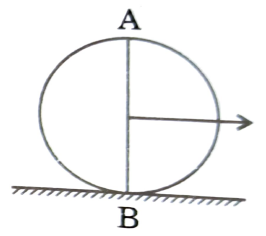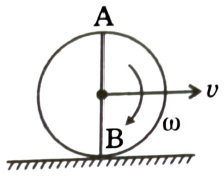Rolling motion
Question

# A uniform solid disc is rolling on a horizontal surface. At a certain instant B is the point of contact and A is at height 2R from ground, where R is radius of disc:Moderate
Solution

## $\stackrel{\to }{\mathrm{L}}=\stackrel{\to }{\mathrm{r}}×\mathrm{M}{\stackrel{\to }{\mathrm{V}}}_{\mathrm{cm}}+{\mathrm{I}}_{\mathrm{cm}}\mathrm{\omega }$Get Instant Solutions